9.6 SPM Practice (Long Questions)

Question 9 (12 marks):
(a) Diagram 9.1 shows point P (5, 1) on a Cartesian plane.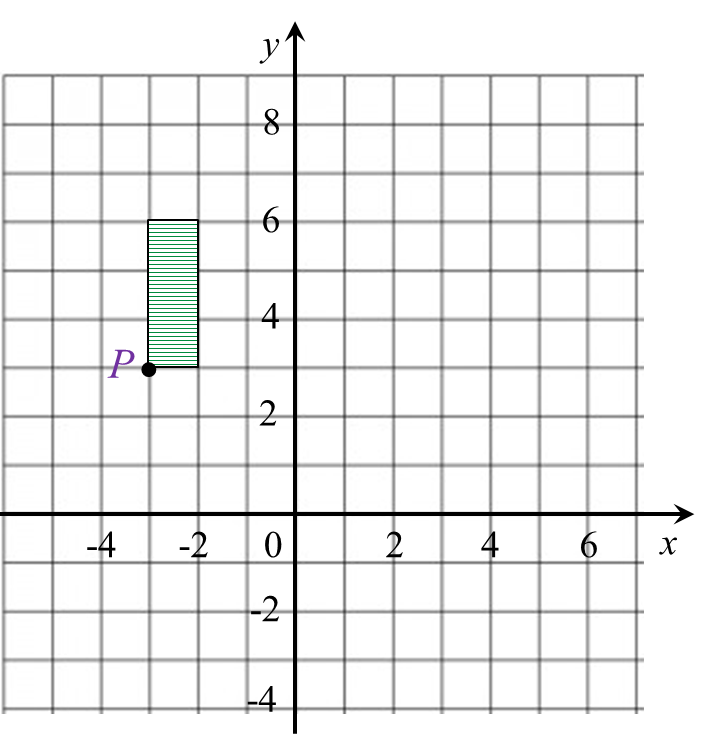Diagram 9.1

Transformation T is a translation
Transformation S is an enlargement about the centre (–5, 2) with a scale factor 2.
State the coordinates of the image of point P under the following transformations:
(i) T2,
(ii) TS.

(b) Diagram  9.2 shows geometrical shapes KLMNP, KSRQP and KTUVW drawn on a Cartesian plane.Diagram 9.2

(i)
KTUVW is the image of KLMNP under the combined transformation YZ.
Describe, in full, the transformation:
(a) Z,
(b) Y.

(ii) It is given that KSRQP represents a region of area 30 m2.
Calculate the area, in m2, of the shaded region.

Solution:
(a)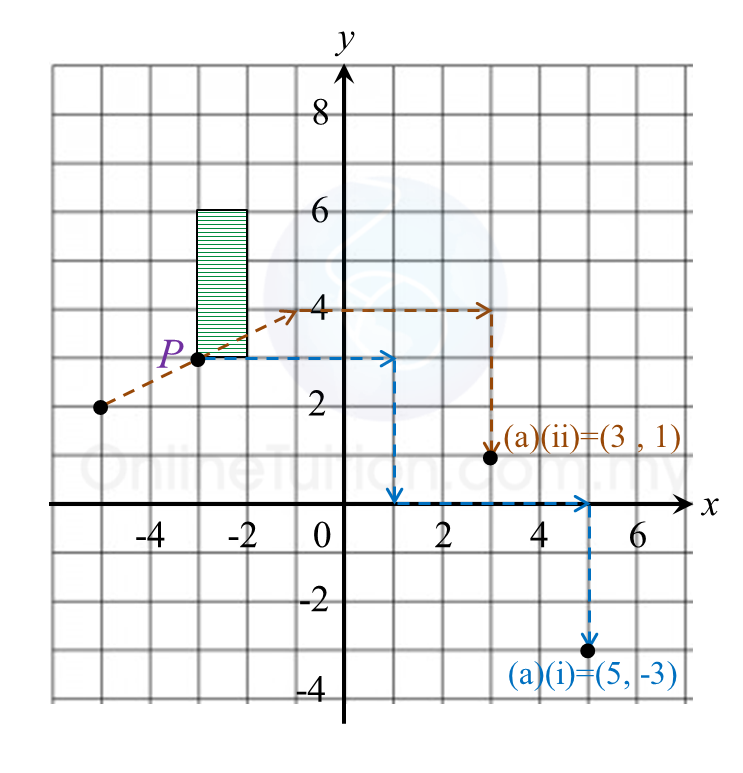(i)
TT = P(–3, 3) → T1 → P’(1, 0) ) → T2 → P’’(5, –3)
(ii) TS =  P (–3, 3) → S → P’(–1, 4) → T → P’’(3, 1)

(b)(i)(a)
Z: Reflection in the line x = 0.

(b)(i)(b)
Y: Enlargement with the centre at (0, 0) and a scale factor of 2.

(b)(ii)
Area of KTUVW = (Scale factor)2 × Area of object
= 22 × 30
= 120 m2

Hence,
= Area KTUVW – Area KSRQP
= 120 – 30
= 90 m2

SPM Practice (Long Questions)

Question 9 (12 marks):
(a) Diagram 7.1 shows K (5, 1) drawn on a Cartesian plane.Diagram  7.1

Transformation T is a translation
Transformation P is a reflection in the line y = 2.
State the coordinates of the images of point K under each of the following transformations:
(i) T2,
(ii) TP.

(b) Diagram 7.2 shows two pentagons KLMNP and QRSTU, drawn on a Cartesian plane.Diagram  7.2

(i)
Pentagon QRSTU  is the image of pentagon KLMNP under the combined transformation WV.
Describe in full the transformation:
(a) V,
(b) W.

(ii)
It is given that pentagon QRSTU represents a region with an area of 90 m2.
Calculate the area, in m2, of pentagon KLMNP.

Solution:
(a)(i)
TT = K(5, 1) → T1 → K’(2, 5) ) → T2 → K’’(–1, 9)
(ii) TP =  K(5, 1) → P → K’(5, 3) → T → K’’(2, 7)

(b)(b)(i)(a)

V: 90o rotation in clockwise direction at point K(3, 7).

(b)(i)(b)
W: Enlargement at point (1, 6) with scale factor 3.

(b)(ii)
Area of QRSTU = (scale factor)2 × area of object
Area of QRSTU = 32 × Area of KLMNP
90 m2 = 9 × Area of KLMNP
Area of KLMNP = 90/9 = 10 m2

SPM Practice (Long Questions)

Question 8:
(a) Diagram 8.1 shows point A and point B marked on a Cartesian plane.Diagram 8.1

Transformation R is a rotation of 90o, clockwise about the centre B.

Transformation T is a translation

State the coordinates of the image of point A under each of the following transformations:
(i) RT,
(ii) R2.

(b)
Diagram 8.2 shows three trapeziums ABCD, PQRS and TUVS, drawn on a Cartesian plane.Diagram 8.2

(i)
Trapezium PQRS is the image of trapezium ABCD under the combined transformation MN.
Describe in full, the transformation:
(a) N,
(b) M.

(ii)
It is given that trapezium ABCD represents a region of area 30 m2.
Calculate the area, in m2, of the shaded region.

Solution:
(a)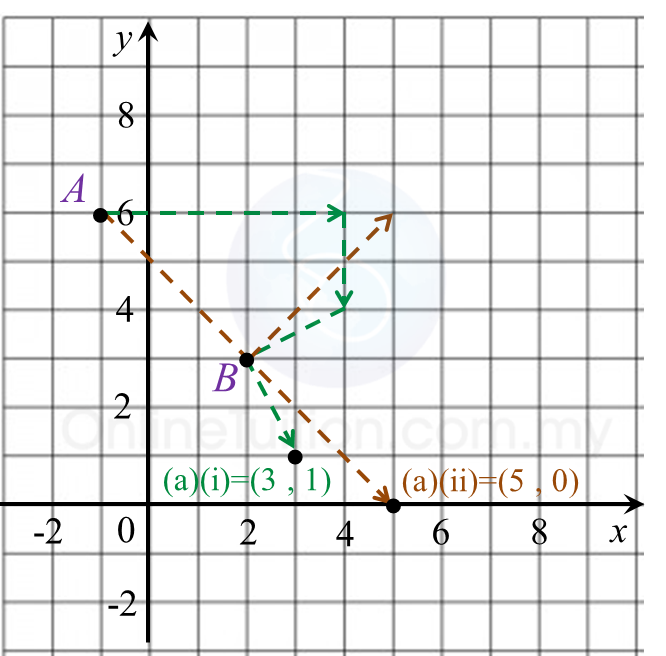(i)
A (–1, 6) → T → (4, 4 ) → R → (3, 1)
(ii) A (–1, 6) → R → (5, 6) → R → (5, 0)

(b)(i)(a)
N is a reflection in the line y = 4.

(b)(ii)(b)
is enlargement of scale factor 2 with centre S (1, 5).

(b)(ii)
Area of PQRS = (scale factor)2 x Area of object ABCD
= 22 x 30
= 120 m2

Therefore,
= Area PQRS – area ABCD
= 120 – 30
= 90 m2

SPM Practice (Long Questions)

Question 7:
Diagram shows the point J(1, 2) and quadrilaterals ABCD and EFGH, drawn on a Cartesian plane.(a)
Transformation U is a rotation of 90o, clockwise about the centre O.
Transformation T is a translation $\left(\begin{array}{l}\text{2}\\ 3\end{array}\right)$
Transformation R is a reflection at the line x = 3.

State the coordinates of the image of point J under each of the following transformations:
(i) RU,
(ii) TR.

(b)
EFGH is the image of ABCD under the combined transformation MN.
Describe in full, the transformation:
(i) N,
(ii) M.

(c)
It is given that quadrilateral ABCD represents a region of area 18 m2.
Calculate the area, in m2, of the shaded region.

Solution:(a)
(i) J (1, 2) → U → (2, –1 ) → R → (4, –1)
(ii) J (1, 2) → R → (5, 2) → T → (7, 5)

(b)(i)
N is a reflection in the line x = 6.

(b)(ii)
M is enlargement of scale factor 3 with centre (8, 7).

(c)
Area EFGH = (scale factor)2 x Area of object ABCD
= 32 x 18
= 162 m2

Therefore,
= Area of EFGH – Area of ABCD
= 162 – 18
= 144 m2

SPM Practice (Long Questions)

Question 6:
(a) Diagram below shows point M marked on a Cartesian plane.Transformation T is a translation $\left(\begin{array}{l}\text{2}\\ 3\end{array}\right)$ and transformation R is an anticlockwise rotation of 90o about the centre O.
State the coordinates of the image of point M under each of the following transformations:
(i) RT,
(ii) TR,

(b) Diagram below shows two hexagons, ABCDEF and JKLANO, drawn on square grids.(i) JKLANO is the image of ABCDEF under the combined transformation VW.
Describe in full the transformation:
(a) W   (b) V

(ii) It is given that quadrilateral ABCDEF represents a region of area 45 m2.
Calculate the area, in m2, of the region represented by the shaded region.

Solution:
(a)(b)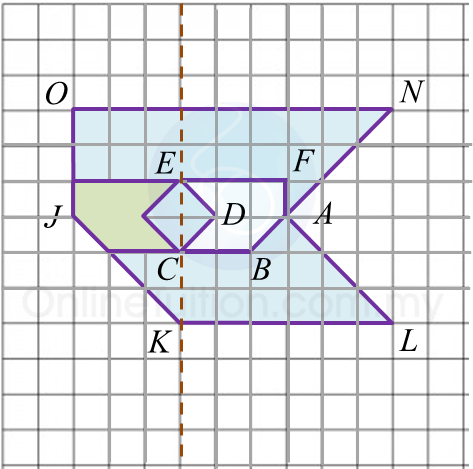(i)(a)
V: A reflection in the line EC.

(i)(b)

(ii)
Area of JKLANO
= 32 x area of ABCDEF
= 9 x 45
= 405 m2

Area of the coloured region
= area of JKLANO – area of ABCDEF
= 405 – 45
= 360 m2

SPM Practice (Long Questions)

Question 5:
(a) Diagram below shows two points, M and N, on a Cartesian plane.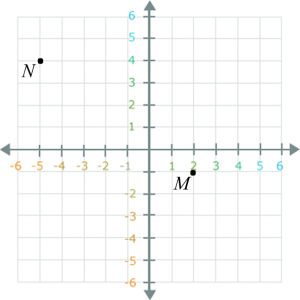Transformation T is a translation and transformation R is an anticlockwise rotation of 90o about the centre (0, 2).
(i) State the coordinates of the image of point M under transformation R.
(ii) State the coordinates of the image of point N under the following transformations:
(a) T2,
(b) TR,

(b) Diagram below shows three pentagons, A, B and C, drawn on a Cartesian plane.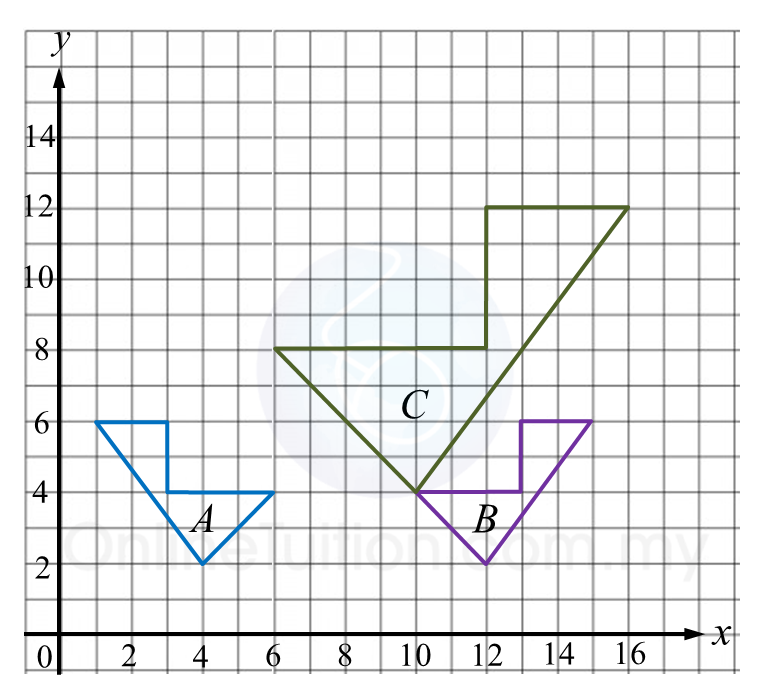(i) C is the image of A under the combined transformation WV.
Describe in full the transformation:
(a) V    (b) W
(ii) Given A represents a region of area 12 m2, calculate the area, in m2, of the region represented by C.

Solution:
(a)(b)(b)(i)(a)
V: A reflection in the line x  = 8

(b)(i)(b)
W: An enlargement of scale factor 2 with centre (14, 0).

(b)(ii)
Area of B = area of A = 12 m2
Area of C = (Scale factor)2 x Area of object
= 22 x area of B
= 22 x 12
= 48 m2

SPM Practice (Long Questions)

Question 4:
Diagram below shows three triangles RPQ, UST and RVQ, drawn on a Cartesian plane.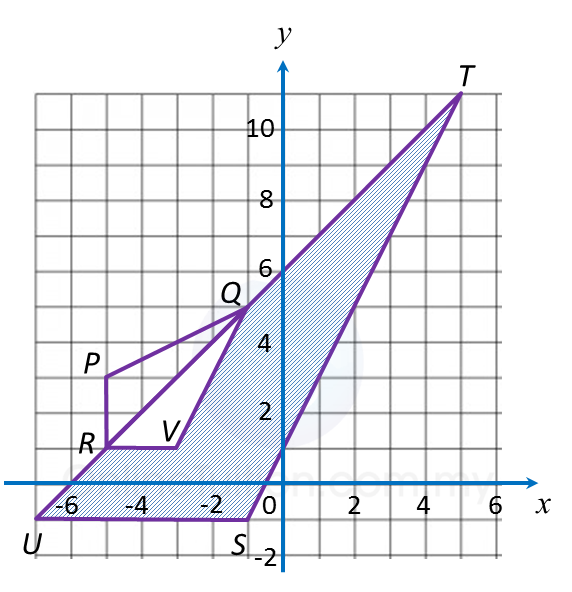(a) Transformation R is a rotation of 90o, clockwise about the centre O.
Transformation T is a translation $\left(\begin{array}{l}2\\ \text{3}\end{array}\right)$ .
State the coordinates of the image of point B under each of the following transformations:
(i) Translation T2,
(ii) Combined transformation TR.

(b)
(i) Triangle UST is the image of triangle RPQ under the combined transformation VW.
Describe in full the transformation:
(a) W   (b) V

(ii) It is given that quadrilateral RPQ represents a region of area 15 m2.
Calculate the area, in m2, of the region represented by the shaded region.

Solution: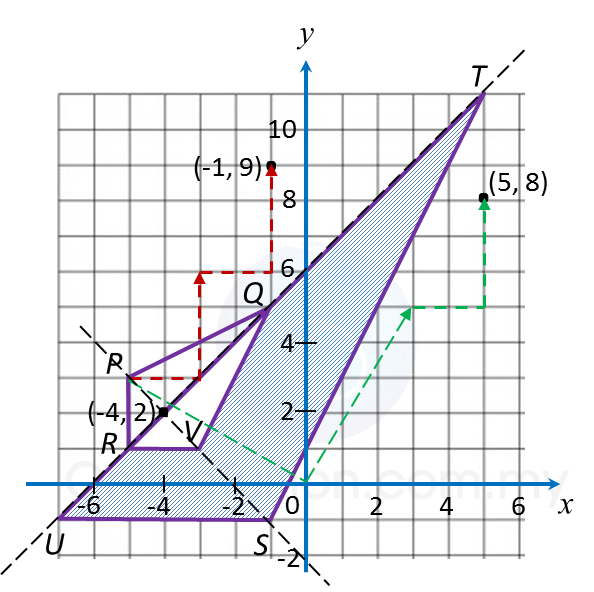(a)
(i) (–5, 3) → T → (–3, 6) ) → T → (–1, 9)
(ii) (–5, 3) → R → (3, 5) → T → (5, 8)

(b)(i)(a)
W: A reflection in the line URQT.

(b)(i)(b)

(b)(ii)
Area of UST = (Scale factor)2 x Area of RPQ
= 32 x area of RPQ
= 32 x 15
= 135 m2

Therefore,
= Area of UST – area of RPQ
= 135 – 15
= 120 m2

SPM Practice (Long Questions)

Transformation III, Long Questions (Question 3)

Question 3:
(a) Transformation P is a reflection in the line x = m.
Transformation is a translation $\left(\begin{array}{l}\text{4}\\ -2\end{array}\right)$ .
Transformation is a clockwise rotation of 90o about the centre (0, 4).

(i) The point (6, 4) is the image of the point ( –2, 4) under the transformation P.
State the value of m

(ii) Find the coordinates of the image of point (2, 8) under the following combined transformations:
(a) T2,
(b) TR.

(b) Diagram below shows trapezium CDFE and trapezium HEFG drawn on a Cartesian plane.(i) HEFG is the image of CDEF under the combined transformation WU.
Describe in full the transformation:
(a) U (b) W

(ii) It is given that CDEF represents a region of area 60 m2.
Calculate the area, in m2, of the region represented by the shaded region.

Solution:
(a)(i)
$\begin{array}{l}\left(6,4\right)\to P\to \left(-2,4\right)\\ m=\frac{6+\left(-2\right)}{2}=2\end{array}$

(a)(ii)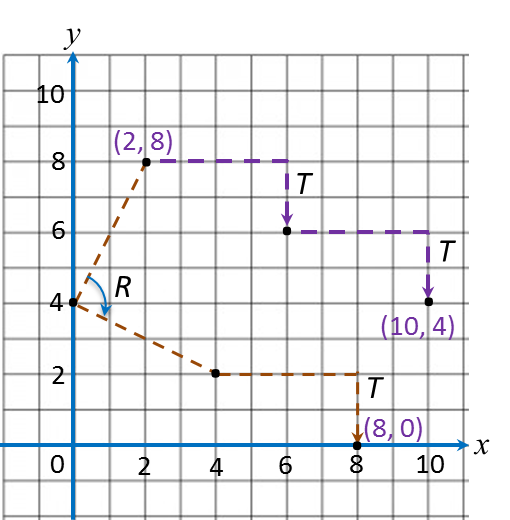(a) (2, 8) → T → (6, 6) → T → (10, 4)
(b) (2, 8) → R → (4, 2) → T → (8, 0)

(b)(i)(a)
U: An anticlockwise rotation of  90oabout the centre A (3, 3).

(b)(i)(b)
$\text{Scale factor}=\frac{HE}{CD}=\frac{4}{2}=2$
W: An enlargement of scale factor 2 with centre B (3, 5).

(b)(ii)
Area of HEFG= (Scale factor)2 × Area of object
= 22 × area of CDEF
= 4 × 60
= 240 m2
Therefore,
= Area of HEFG– area of CDEF
= 240 – 60
= 180 m2

SPM Practice (Long Questions)

Question 2:
(a) Diagram below shows point A and straight line y + x = 5 drawn on a Cartesian plane.Transformation T is a translation
Transformation R is a reflection at the line y + x = 5.

State the coordinates of the image of point A under each of the following transformations:
(i) Transformation T,
(ii) Combined transformation TR.

(b) Diagram below shows pentagons JKLMN, PQRST and PUVWX, drawn on a Cartesian plane.(i) PUVWX is the image of JKLMN under the combined transformation CB.
Describe in full the transformation:
(a) B    (b) C

(ii) It is given that pentagon JKLMN represents a region of area 80 m2 .
Calculate the area, in m2 , of the region represented by the shaded region.

Solution:
(a)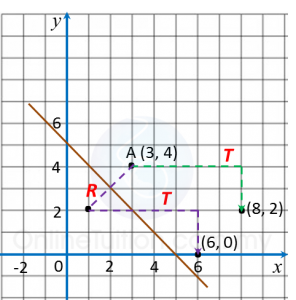(i)
(3, 4) → T → (8, 2)
(ii)
(3, 4) → R → (1, 2) → T → (6, 0)

(b)(b)(i)(a)
B:
A clockwise rotation of  90o about the centre (0, 2).

(b)(i)(b)

(b)(ii)
Area of PQRST = Area of JKLMN
= 80 m2

SPM Practice (Long Questions)

Further Practice:
Transformation III, Long Questions (Question 1)

Question 1:
(a) Transformation is a translation $\left(\begin{array}{l}-4\\ \text{}2\end{array}\right)$ and transformation P is an anticlockwise rotation of 90oabout the centre (1, 0).

State the coordinates of the image of point (5, 1) under each of the following transformation:
(i) Translation T,
(ii) Rotation P,
(iii) Combined transformation T2.

(b) Diagram below shows three quadrilaterals, ABCD, EFGH and JKLM, drawn on a Cartesian plane.(i) JKLM is the image of ABCD under the combined transformation VW.
Describe in full the transformation:
(a) W   (b) V

(ii) It is given that quadrilateral ABCD represents a region of area 18 m2.
Calculate the area, in m2, of the region represented by the shaded region.

Solution:
(a)(b)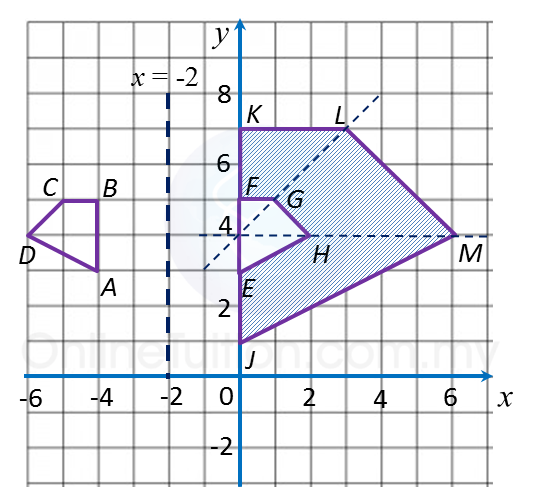(i)(a)
W: A reflection in the line x = –2

(i)(b)
V: An enlargement of scale factor 3 with centre (0, 4).

(b)(ii)
Area of EFGH = area of ABCD = 18 m2
Area of JKLM = (Scale factor)2 × Area of object
= 32 × area of EFGH
= 32 × 18
= 162 m2
Therefore,